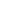# Solve addition problems using ten as a unit, and write two-step solutions.

EngageNY 60 min(s)
In this lesson, students solve addition problems that result in a sum composed of 1 ten and some ones using the "make a ten" strategy, and record their thinking with two equations. For example, they recognize how to add 8 + 4 with the equations 8 + 2 = 10 and 10 + 2 = 14.